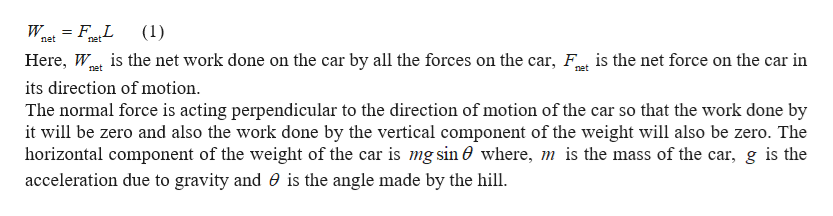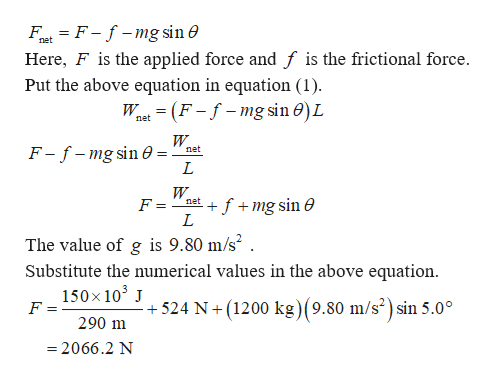# A 1200-kg car is being driven up a 5.0° hill. The frictional force is directed opposite to the motion of the car and has a magnitude of f = 524 N. A force →FF→ is applied to the car by the road and propels the car forward. In addition to these two forces, two other forces act on the car: its weight −→WW→ and the normal force →FNF→N directed perpendicular to the road surface. The length of the road up the hill is 290 m. What should be the magnitude of →FF→, so that the net work done by all the forces acting on the car is +150 kJ?

Question
146 views

A 1200-kg car is being driven up a 5.0° hill. The frictional force is directed opposite to the motion of the car and has a magnitude of f = 524 N. A force →FF→ is applied to the car by the road and propels the car forward. In addition to these two forces, two other forces act on the car: its weight −→WW→ and the normal force →FNF→N directed perpendicular to the road surface. The length of the road up the hill is 290 m. What should be the magnitude of →FF→, so that the net work done by all the forces acting on the car is +150 kJ?

check_circle

Step 1

The equation for the net work done on the car by all the forces on the car is given byhelp_outlineImage Transcriptionclose= F_L (1) net net Here, W is the net work done on the car by all the forces on the car, F is the net force on the car in its direction of motion The normal force is acting perpendicular to the direction of motion of the car so that the work done by it will be zero and also the work done by the vertical component of the weight will also be zero. The horizontal component of the weight of the car is mg sin 0 where, m is the mass of the car, g is the acceleration due to gravity and is the angle made by the hill net fullscreen
Step 2

The equation for the net force on the car in...help_outlineImage TranscriptioncloseFt F-fmg sin 0 Here, F is the applied force and f is the frictional force Put the above equation in equation (1) net Wnet(F-f-mg sin 0)L W F-f-mg sin e L net W net fmg sin e L F = The value of g is 9.80 m/s2 . Substitute the numerical values in the above equation 150x 103 +524 N+ (1200 kg)(9.80 m/s2) sin 5.0° F = 290 m 2066.2 N fullscreen

### Want to see the full answer?

See Solution

#### Want to see this answer and more?

Solutions are written by subject experts who are available 24/7. Questions are typically answered within 1 hour.*

See Solution
*Response times may vary by subject and question.
Tagged in

### Physics